Cubes And Dice Quiz II, Non Verbal Reasoning

# Cubes And Dice Quiz II, Non Verbal Reasoning - SSC CGL

Test Description

## 25 Questions MCQ Test Non Verbal Reasoning - Cubes And Dice Quiz II, Non Verbal Reasoning

Cubes And Dice Quiz II, Non Verbal Reasoning for SSC CGL 2023 is part of Non Verbal Reasoning preparation. The Cubes And Dice Quiz II, Non Verbal Reasoning questions and answers have been prepared according to the SSC CGL exam syllabus.The Cubes And Dice Quiz II, Non Verbal Reasoning MCQs are made for SSC CGL 2023 Exam. Find important definitions, questions, notes, meanings, examples, exercises, MCQs and online tests for Cubes And Dice Quiz II, Non Verbal Reasoning below.
Solutions of Cubes And Dice Quiz II, Non Verbal Reasoning questions in English are available as part of our Non Verbal Reasoning for SSC CGL & Cubes And Dice Quiz II, Non Verbal Reasoning solutions in Hindi for Non Verbal Reasoning course. Download more important topics, notes, lectures and mock test series for SSC CGL Exam by signing up for free. Attempt Cubes And Dice Quiz II, Non Verbal Reasoning | 25 questions in 50 minutes | Mock test for SSC CGL preparation | Free important questions MCQ to study Non Verbal Reasoning for SSC CGL Exam | Download free PDF with solutions
 1 Crore+ students have signed up on EduRev. Have you?
Cubes And Dice Quiz II, Non Verbal Reasoning - Question 1

### Three positions of a dice are given. Based on them find out which number is found opposite the number 2 in the given cube.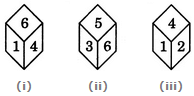Detailed Solution for Cubes And Dice Quiz II, Non Verbal Reasoning - Question 1

From figures (i) and (ii), we conclude that the numbers 1, 4, 3 and 5 lie adjacent to the number 6. Clearly, the number 2 lies opposite 6 and conversely 6 lies opposite 2.

Cubes And Dice Quiz II, Non Verbal Reasoning - Question 2

### The six faces of a dice have been marked with alphabets A, B, C, D, E and F respectively. This dice is rolled down three times. The three positions are shown as: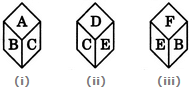Find the alphabet opposite A.

Detailed Solution for Cubes And Dice Quiz II, Non Verbal Reasoning - Question 2

From figures (ii) and (iii), we conclude that the alphabets C, D, B and F appear adjacent to the alphabet E. Therefore, the alphabet A appears opposite E. Conversely, E appears opposite A.

Cubes And Dice Quiz II, Non Verbal Reasoning - Question 3

### A dice is thrown four times and its four different positions are shown below. Find the number on the face opposite the face showing 2.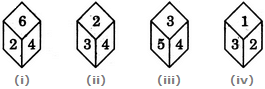Detailed Solution for Cubes And Dice Quiz II, Non Verbal Reasoning - Question 3

From figures (i), (ii) and (iv) We conclude that 6, 4, 3 and 1 lie adjacent to 2. Hence, 5 must lie opposite 2.

Cubes And Dice Quiz II, Non Verbal Reasoning - Question 4

A dice is rolled twice and the two positions are shown in the figure below. What is the number of dots at the bottom face when the dice is in position (i)?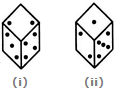Detailed Solution for Cubes And Dice Quiz II, Non Verbal Reasoning - Question 4
From figures (i) and (ii) we conclude that 3, 4, 1 and 5 dots appear adjacent to 2 dots. Therefore, 6 dots must appear opposite 2 dots. Since, there are 2 dots on the top face when the dice is in position (i), therefore, the number of dots at the bottom face must be 6.
Cubes And Dice Quiz II, Non Verbal Reasoning - Question 5

Two positions of a dice are shown. When 4 is at the bottom, what number will be on the top?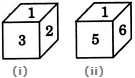Detailed Solution for Cubes And Dice Quiz II, Non Verbal Reasoning - Question 5
From figures (i) and (ii), we conclude that 2, 3, 5 and 6 he adjacent to 1. Therefore, 4 lies opposite 1. Hence, when 4 is at the bottom, then1 must be on the top.
Cubes And Dice Quiz II, Non Verbal Reasoning - Question 6

Below are depicted the three different positions of a dice. Find the number of dots on the face opposite to the face with one dot.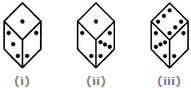Detailed Solution for Cubes And Dice Quiz II, Non Verbal Reasoning - Question 6

From figures (i), (ii) and (iii), we conclude that 1, 3, 5 and 6 dots appear adjacent to the face with 2 dots. Therefore, 4 dots will appear opposite to 2 dots. Now, from figures (i) and (ii), we conclude that 2, 3 and 5 dots appear adjacent to 1 dot Therefore, either 4 or 6 dots will appear opposite to 1 dot. But since, 4 dots appear opposite to 2 dots it follows that 6 dots will appear opposite 1 dots.

Cubes And Dice Quiz II, Non Verbal Reasoning - Question 7

A dice is numbered from 1 to 6 in different ways.

If 1 is adjacent to 2, 3 and 5, then which of the following statements is necessarily true?

Detailed Solution for Cubes And Dice Quiz II, Non Verbal Reasoning - Question 7
If 1 is adjacent to 2, 3 and 5, then either 4 or 6 lies opposite to 1. So, the numbers 4 and 6 cannot lie opposite to each other. Hence, 4 necessarily lies adjacent to 6.
Cubes And Dice Quiz II, Non Verbal Reasoning - Question 8

Two positions of a parallelepiped are shown below. When the number 3 will be on the top side, then which number will be at the bottom?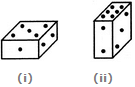Detailed Solution for Cubes And Dice Quiz II, Non Verbal Reasoning - Question 8
The number 2 is common to both the figures. We assume the parallelepiped in fig. (ii) to be rotated so that 2 appears at the same position as in fig. (i) i.e. on the RHS face and the numbers 6 and 3 move to the faces hidden behind the numbers 1 and 5 respectively [in fig. (i)]. Then, the combined figure will have 1 opposite 6 and 5 opposite 3. Thus, when 3 will be on the top, then 5 will appear at the bottom.
Cubes And Dice Quiz II, Non Verbal Reasoning - Question 9

If the total number of dots on opposite faces of a cubical block is always 7, find the figure which is correct.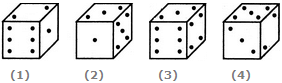Detailed Solution for Cubes And Dice Quiz II, Non Verbal Reasoning - Question 9

Since the total number of dots on opposite faces is always 7, therefore, 1 dot appears opposite 6 dots, 2 dots appear opposite 5 dots and 3 dots appear opposite 4 dots.

Cubes And Dice Quiz II, Non Verbal Reasoning - Question 10

What number is opposite 3 in the figure shown below? The given two positions are of the same dice whose each surface bears a number among 1, 2, 3, 4, 5 and 6.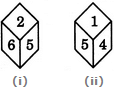Detailed Solution for Cubes And Dice Quiz II, Non Verbal Reasoning - Question 10
From figures (i) and (ii), we conclude that 2, 6, 1 and 4 appear adjacent to 5. Therefore, 3 must appear opposite 5, Conversely, the number opposite 3 is 5.
Cubes And Dice Quiz II, Non Verbal Reasoning - Question 11

Two positions of a block are given below. When 1 is at the top, which number will be at the bottom?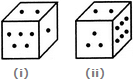Detailed Solution for Cubes And Dice Quiz II, Non Verbal Reasoning - Question 11
Number 2 is common to the two positions of the dice. We assume the dice in fig. (ii) to be rotated so that 2 remains on the top face (i.e. face V as per activity 1) and the numbers 3 and 6 move to the faces hidden behind 5 and 1 respectively [in fig. (i)]. Then, clearly, 5 lies opposite 3 and 6 lies opposite 1. Hence, when 1 is at the top, then 6 will be at the bottom.
Cubes And Dice Quiz II, Non Verbal Reasoning - Question 12

What will be the number at the bottom, if 5 is at the top; the two positions of the dice being as given below: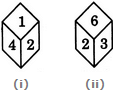Detailed Solution for Cubes And Dice Quiz II, Non Verbal Reasoning - Question 12
From figures (i) and (ii), it is clear that 4, 1, 3 and 6 he adjacent to 2. Therefore, 5 must lie opposite 2. Thus, if 5 is at the top, then 2 must be at the bottom.
Cubes And Dice Quiz II, Non Verbal Reasoning - Question 13

Two positions of a dice are shown below. Identify the number at the bottom when the top is '3'?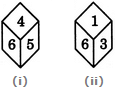Detailed Solution for Cubes And Dice Quiz II, Non Verbal Reasoning - Question 13
The number 6 is common to both the positions of the dice. We assume the dice in fig. (ii) to be rotated so that 6 remains on the FR-LH face (i. e. on face IV as per activity 1) and the numbers 1 and 3 move to the faces hidden behind 4 and 5 respectively [in fig. (i)]. Then, 5 lies on FR-RH face (i.e. face I), 4 lies on Top face {i.e. face V), 3 lies on RR-LH face (i.e. face III) and 1 lies on Bottom face (i.e. face VI). Thus, 3 lies opposite 5. Hence, when the top is 3, then the number at the bottom is 5.
Cubes And Dice Quiz II, Non Verbal Reasoning - Question 14

Three different positions X, Y and Z of a dice are shown in the figures given below. Which number lies at the bottom face in position X?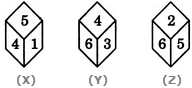Detailed Solution for Cubes And Dice Quiz II, Non Verbal Reasoning - Question 14

From positions X and Y we conclude that 1, 5, 6 and 3 lie adjacent to 4. Therefore, 2 must lie opposite 4. From positions Y and Z we conclude that 4, 3, 2 and 5 lie adjacent to 6. Therefore, 1 must lie opposite 6. Thus, 2 lies opposite 4, 1 lies opposite 6 and consequently 5 lies opposite 3.

As analysed above, the number on the face opposite 5 is 3. In position X, since 5 lies on the top, therefore 3 must lie at the bottom face.

Cubes And Dice Quiz II, Non Verbal Reasoning - Question 15

A dice is numbered from 1 to 6 in different ways.

If 2 is opposite to 3 and adjacent to 4 and 6, then which of the following statements is necessarily true?

Detailed Solution for Cubes And Dice Quiz II, Non Verbal Reasoning - Question 15
If 2 is opposite to 3, then 1 cannot lie opposite to either of the two numbers - 2 or 3. Hence, 1 is necessarily adjacent to both 2 and 3.
Cubes And Dice Quiz II, Non Verbal Reasoning - Question 16

Two positions of a dice are shown below. When number 1 is on the top, what number will be at the bottom?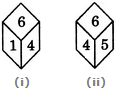Detailed Solution for Cubes And Dice Quiz II, Non Verbal Reasoning - Question 16
Number 6 is common to both the positions of the dice. We assume the dice in fig. (ii) to be rotated so that 6 remains on the top face (i.e. face V as per activity 1) and the number 4 in fig. (ii) moves to the FR-RH face (i.e. face I) as in fig. (i), then 5 will move to the RR-RH face (i.e. face II). Clearly, 5 (which lies on face II) and 1 (which lies on face IV) will be opposite to each other. So, when 1 is on the top, then 5 will be at the bottom.
Cubes And Dice Quiz II, Non Verbal Reasoning - Question 17

Two positions of a cube are shown below. When the number 4 will be at the bottom, then which number will be at the top?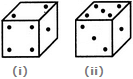Detailed Solution for Cubes And Dice Quiz II, Non Verbal Reasoning - Question 17
Number 1 is common to both the positions of the dice. We assume the dice in fig. (ii) to be rotated so that 1 remains on the RHS face (i.e. face II as per activity 1) and the numbers 5 and 3 move to the faces hidden behind 2 and 4 respectively (in fig. (i). Then, clearly 3 lies opposite 4. Hence, when 4 is at the bottom then 3 mist lie on the top.
Cubes And Dice Quiz II, Non Verbal Reasoning - Question 18

Two positions of a block are shown below: When six is at the bottom, what number will be at the top?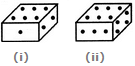Detailed Solution for Cubes And Dice Quiz II, Non Verbal Reasoning - Question 18
From figures (i) and (ii) we conclude that the number 1, 2, 3 and 4 appear adjacent to 6. Thus, the number 5 will appear opposite 6. Therefore, when six is at the bottom, then 5 will be at the top.
Cubes And Dice Quiz II, Non Verbal Reasoning - Question 19

Two positions of a dice are shown below: When 2 is at the bottom, what number will be at the top?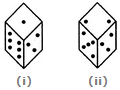Detailed Solution for Cubes And Dice Quiz II, Non Verbal Reasoning - Question 19
Number 3 is common to both the figures (i) and (ii). The dice in fig. (ii) is assumed to be rotated so that 3 remains on the FR-RH face (i.e. face I as per activity 1) and the numbers 5 and 2 move to the faces hidden behind the numbers 6 and 1 respectively [in fig. (i)]. Thus, the combined figure will have 3 on FR-RH face (i.e. face I), 5 on RR-RH face (i.e. face II), 2 on Bottom face (i.e. face VI), 1 on the Top face (i.e. face V) and 6 on FR-LH face (i.e. face IV). Clearly, 2 lies opposite 1. Hence, when 2 is at the bottom, then 1 will be at the top.
Cubes And Dice Quiz II, Non Verbal Reasoning - Question 20

A dice is numbered from 1 to 6 in different ways.

If 1 is opposite to 5 and 2 is opposite to 3, then

Detailed Solution for Cubes And Dice Quiz II, Non Verbal Reasoning - Question 20
If 1 is opposite to 5 and 2 is opposite to 3, then 4 definitely lies opposite to 6. Therefore, 2 cannot lie opposite to any of the two numbers - 4 or 6. Hence, 2 necessarily lies adjacent to both 4 and 6.
Cubes And Dice Quiz II, Non Verbal Reasoning - Question 21

Three different positions X, Y and Z of a dice are shown in the figures given below. Which numbers are hidden behind the numbers 6 and 5 in the position Z?Detailed Solution for Cubes And Dice Quiz II, Non Verbal Reasoning - Question 21

From positions X and Y we conclude that 1, 5, 6 and 3 lie adjacent to 4. Therefore, 2 must lie opposite 4. From positions Y and Z we conclude that 4, 3, 2 and 5 lie adjacent to 6. Therefore, 1 must lie opposite 6. Thus, 2 lies opposite 4, 1 lies opposite 6 and consequently 5 lies opposite 3.

As analysed above, the number opposite 6 is 1 and the number opposite 5 is 3. Therefore, the numbers hidden behind the numbers 6 and 5 in position Z (these are the numbers opposite 5 and 6 respectively) are 1 and 3.

Cubes And Dice Quiz II, Non Verbal Reasoning - Question 22

Four positions of a dice are shown below. What number must be at the bottom face when the dice is in the position as shown in the figure(iii)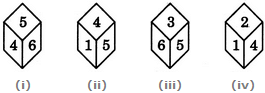Detailed Solution for Cubes And Dice Quiz II, Non Verbal Reasoning - Question 22

From figures (i), (ii), (iv) we conclude that 5, 6, 1 and 2 lie adjacent to 4. Hence, 3 must lie opposite 4 and vice-versa. In fig. (iii), 3 is at the top and consequently 4 must lie at the bottom face.

Cubes And Dice Quiz II, Non Verbal Reasoning - Question 23

Two positions of a block are shown below. When 2 is at the bottom, which number will be at the top?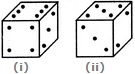Detailed Solution for Cubes And Dice Quiz II, Non Verbal Reasoning - Question 23

Number 3 is common to the two positions of the block. We assume the block in fig. (ii) to be rotated so that 3 appears at the same position as in fig. (i) i.e. on RHS face (i.e. on face II as per activity 1) and the numbers 5 and 2 move to the faces hidden behind the numbers 4 and 6 respectively [in fig. (i)]. Thus, the combined figure will have 3 on RHS face (i.e. face II), 4 on the Front face (i.e. face I), 6 on the Top face (i.e. face V), 5 on the Rear face (i.e. face III) and 2 on the Bottom face (i.e. face VI). Clearly, when 2 is at the bottom; then 6 is at the top.

Cubes And Dice Quiz II, Non Verbal Reasoning - Question 24

Three different positions X, Y and Z of a dice are shown in the figures given below. Which number lies at the bottom face in position Y?Detailed Solution for Cubes And Dice Quiz II, Non Verbal Reasoning - Question 24

From positions X and Y we conclude that 1, 5, 6 and 3 lie adjacent to 4. Therefore, 2 must lie opposite 4. From positions Y and Z we conclude that 4, 3, 2 and 5 lie adjacent to 6. Therefore, 1 must lie opposite 6. Thus, 2 lies opposite 4, 1 lies opposite 6 and consequently 5 lies opposite 3.

As analysed above, the number on the face opposite 4 is 2. In position Y, since 4 lies on the top, therefore 2 must lie at the bottom face.

Cubes And Dice Quiz II, Non Verbal Reasoning - Question 25

What is the opposite 3, if four different positions of dice are as shown below?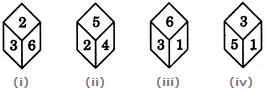Detailed Solution for Cubes And Dice Quiz II, Non Verbal Reasoning - Question 25

From figures (i), (iii) and (iv), we conclude that 2, 6, 1. and 5 appear adjacent to 3. Clearly, 4 will appear opposite 3.

## Non Verbal Reasoning

40 tests
Information about Cubes And Dice Quiz II, Non Verbal Reasoning Page
In this test you can find the Exam questions for Cubes And Dice Quiz II, Non Verbal Reasoning solved & explained in the simplest way possible. Besides giving Questions and answers for Cubes And Dice Quiz II, Non Verbal Reasoning, EduRev gives you an ample number of Online tests for practice

40 tests